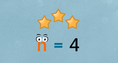# Solving Algebraic Equations II

### DESCRIPTION

Is solving equations tricky? If you know how to isolate a variable, you're halfway there. The other half? Don't do anything to upset the balance of an equation. Join our plucky variable friend as he encounters algebraic equations and a (sometimes grumpy) equal sign. With a little practice, you'll find that solving equations isn't tricky at all.

Full Lesson Info